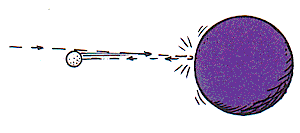# Conceptual Physics: IIYou have a friend who says that: "After a golf ball collides with a bowling ball at rest, although the speed gained by the bowling ball is very small, its momentum exceeds the initial momentum of the golf ball." Your friend further asserts that this is related to the "negative" momentum of the golf ball after collision.

Your another friend says this is hogwash, and thinks that the momentum conservation would be violated. Which friend do you agree with, and why?

A. The first friend:

We have $mv_{before}=mv_{after}.$ The bowling ball's momentum exceeds that of the initial momentum of the golf ball, but this is to account for the "negative" momentum of the golf ball. The opposite momenta cancel, and the Law of Conservation of Momentum is not violated.

B. The second friend:

By the Law of Conservation of Momentum, $mv_{before}=mv_{after}$. Since the momentum of the bowling ball was initially zero, the momentum of the bowling ball cannot exceed the initial momentum of the golf ball because that would imply the system has gained momentum.

C. The first friend:

By the Law of Conservation of Momentum, $mv_{before}=mv_{after}$. Since the initial momentum of the golf ball was in one direction, after the collision, it went in another direction, thus gaining negative momentum with respect to the bowling ball's momentum. To offset this negative momentum, the bowling ball's momentum must be greater than the initial momentum of the golf ball.

D. The second friend:

Not only would the collision violate the Law of Conservation of Momentum, $mv_{before}=mv_{after}$, but it would violate the principle of elastic collisions. The "negative" momentum of the golf ball is impossible, for then it would not be transfer its initial momentum to the bowling ball (think billiard balls: when one ball strikes another, its momentum is transferred to the ball receiving the hit; but the striking billiard ball never changes its direction of motion).Details and Assumptions

1. For simplicity purposes, you may assume the interaction takes place in 1 dimensional space (linear motion), and 1 dimension of time (and 1 for every other cosmic parameter beyond 7D hyperverses).

2. The golf ball and the bowling ball are the only two objects in the universe before the interaction, during the interaction, and after.

###### Problem credit: Conceptual Physics: Special Edition Series - Paul G. Hewitt.
×

Problem Loading...

Note Loading...

Set Loading...